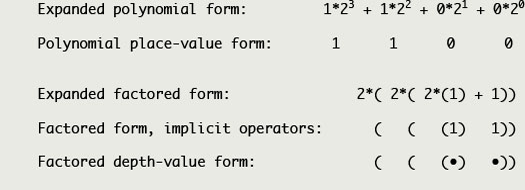1.6.2 Circle Numbers home page Circle numbers represent magnitude using a depth-value notation that supports a maximally factored form, as opposed to conventional Hindu-Arabic numbers that use place-value to represent magnitude in a polynomial form. Both use a uniform base; both support an arbitrary choice of base value. The factored circle form provides distinct computational advantages, at a slight cost to ease of readability. Maximally factored numbers are clumsy when expressed in conventional notation, but quite elegant when expressed in a boundary notation. More fundamentally, boundary notation shows that the foundational properties of group theory are largely syntactic. boundary math numerics number systems ∆ circle numbers graph numbers links site structure Conventional polynomial and factored boundary forms of binary twelve:Three animations follow. They each display the same materials, but with varying degrees of condensation. CIRCLE NUMBERS ANIMATION (complete version, 11 minutes) CIRCLE NUMBERS ANIMATION (condensed version, 4 minutes) ADDITION AND MULTIPLICATION OF CIRCLE NUMBERS (short version, 2:20 minutes)